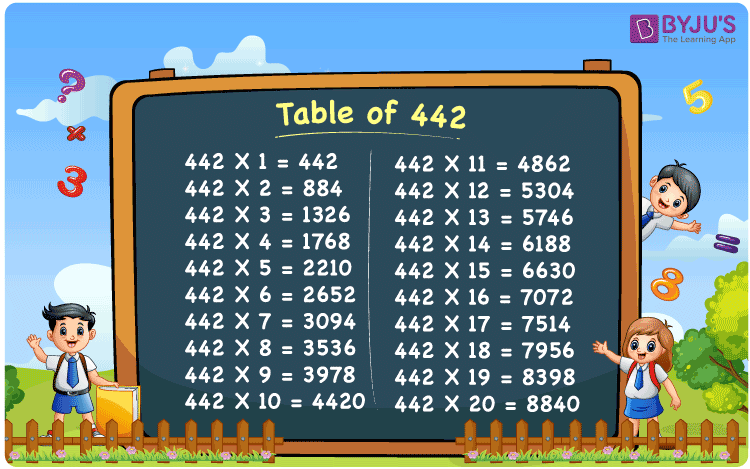Checkout JEE MAINS 2022 Question Paper Analysis : Checkout JEE MAINS 2022 Question Paper Analysis :

# Table of 442

The Table of 442 is given here to help students quickly check the times table of 442 up to 20. The multiplication table of 442 given below is in tabular format, and a PDF is also provided to download. They can download the PDF and use the image as a flashcard, which will help them memorise the table of 442.

## Table of 442 Chart

The table chart of 442 is helpful for quickly go through the values of multiples of 442. The printable image for the table of 442 can be used as a flashcard, and is valuable for doing mental maths and solving problems for competitive exams.## What is the 442 Times Table?

The 442 times table is the multiplication of 442 with any natural number. The 442 times table can be obtained both by multiplication and by adding 442 repeatedly. The below chart shows the multiplication table of 442, in both multiplication and addition form.

 442×1 = 442 442 442×2 = 884 442 + 442 = 884 442×3 = 1326 442 + 442 + 442 = 1326 442×4 = 1768 442 + 442 + 442 + 442 = 1768 442×5 = 2210 442 + 442 + 442 + 442 + 442 = 2210 442×6 = 2652 442 + 442 + 442 + 442 + 442 + 442 = 2652 442×7 = 3094 442 + 442 + 442 + 442 + 442 + 442 + 442 = 3094 442×8 = 3536 442 + 442 + 442 + 442 + 442 + 442 + 442 + 442 = 3536 442×9 = 3978 442 + 442 + 442 + 442 + 442 + 442 + 442 + 442 + 442 = 3978 442×10 = 4420 442 + 442 + 442 + 442 + 442 + 442 + 442 + 442 + 442 + 442 = 4420

## Multiplication Table of 442

Given below are the first 20 multiples of 442.

 442 × 1 = 442 442 × 2 = 884 442 × 3 = 1326 442 × 4 = 1768 442 × 5 = 2210 442 × 6 = 2652 442 × 7 = 3094 442 × 8 = 3536 442 × 9 = 3978 442 × 10 = 4420 442 × 11 = 4862 442 × 12 = 5304 442 × 13 = 5746 442 × 14 = 6188 442 × 15 = 6630 442 × 16 = 7072 442 × 17 = 7514 442 × 18 = 7956 442 × 19 = 8398 442 × 20 = 8840

## Solved Example on the Table of 442

Example 1:

What is 3 times the third multiple of 442?

Solution:

3 times the third multiple of 442 = (442 × 3) × 3 = 442 × 9

= 3978.

## Frequently Asked Questions on Table of 442

### What is the 442 times table?

The 442 times table is the multiplication table of 442 with natural numbers. For example, 442 × 1 = 442, 442 × 2 = 884, 442 × 3 = 1326, 442×4 = 1768, 442×5 = 2210 and so on.

### What times table has 442?

442 comes in the times tables of 1, 2, 13, 17, 26, 34, 221 and 442.

### Is 442 a prime number?

No, 442 is a composite number.